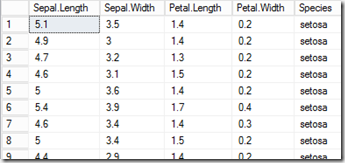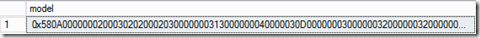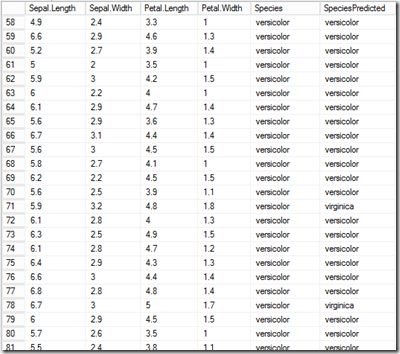## Friday, November 6, 2015

### Using R in SQL Server 2016 CTP 3 to train and query a predictive model

SQL Server 2016 CTP 3
One of the exciting features of SQL Server 2016 is the R integration based on Revolution R Enterprise. The online documentation contains detailed information about the installation steps but only a few examples about how to use this new feature.
The installation process is explained here: https://msdn.microsoft.com/en-us/library/mt604883.aspx
• Install Revolution R Open for R Enterprise
• Install Revolution R Enterprise
• Register components in SQL Server and configure SQL Server to allow external scripts
The online documentation also contains two examples of how to call an R function from within SQL Server. The first example just returns a data frame from R (in this case the pre-defined iris data set):
CREATE PROC get_iris_dataset
AS
BEGIN
EXEC sp_execute_external_script
@language = N'R'
, @script = N'iris_data <- iris;'
, @input_data_1 = N''
, @output_data_1_name = N'iris_data'
WITH RESULT SETS (("Sepal.Length" float not null, "Sepal.Width" float not null,"Petal.Length" float not null, "Petal.Width" float not null, "Species" varchar(100)));
END;

You can see the call to the external script here. I changed the text color of the R statement to blue so it’s easier to be found within the SQL code. We have no input data for this simple case but return a table using the WITH RESULT SETS clause of the stored procedure.
Executing the procedure get_iris_dataset just shows the data from the R script in SQL Server. Here are the first rows of the result set.I want to use this data as my training data, so I load the data into a SQL Server table. Usually, your training data would already be in a table so you wouldn’t need the procedure from above.
create table iris_data
(
"Sepal.Length" float not null,
"Sepal.Width" float not null,
"Petal.Length" float not null,
"Petal.Width" float not null,
"Species" varchar(100)
)
GO
INSERT INTO iris_data Exec get_iris_dataset
GO

So, this copies the data from R into a SQL Server table. Next, I’d like to train an R model based on this data. Again, this code can be found in the documentation:
CREATE PROC generate_iris_model
AS
BEGIN
EXEC sp_execute_external_script
@language = N'R'
, @script = N'
library(e1071);
irismodel <-naiveBayes(iris_data[,1:4], iris_data[,5]);
trained_model <- data.frame(payload = as.raw(serialize(irismodel, connection=NULL)));
'
, @input_data_1 = N'select "Sepal.Length", "Sepal.Width", "Petal.Length", "Petal.Width", "Species" from iris_data'
, @input_data_1_name = N'iris_data'
, @output_data_1_name = N'trained_model'
WITH RESULT SETS ((model varbinary(max)));
END;

Again, the R code is in blue. If you haven’t installed the library e1071 in R you have to do so before running this procedure. To so, open the R console and run install.packages(e1071) as described here.
The interesting thing about this procedure is, that we actually return the trained model as a varbinary object. In R the object class is “naiveBayes” but here it is serialized to a raw data frame that is returned from the function. You could also save the model in R (using the ‘save’ command) but it’s still interesting to see you we can store the model in SQL Server. To do so, I create a simple table and persist the trained model there:
CREATE TABLE [dbo].[model](
[model] [varbinary](max) NULL
)
GO
insert into model exec generate_iris_model
GOSo now we’re getting to the more interesting part of the game which took me some time to figure out. If we want to query the existing model, we have to de-serialize it and use a prediction function. For simplicity I’m using the same data set (iris) again to see how well the model performs on known data. First we load the model into a variable (again varbinary) and then we pass the model to the external script together with the data on which we like to do our prediction. Here is the final code:
declare @model varbinary(MAX)
select @model=model from model

EXEC sp_execute_external_script
@language = N'R'
, @script = N'
library(e1071)
model <-unserialize(as.raw(iris_model))
pred<-predict(model, iris_data)
result<-cbind(iris_data, pred)
'
, @input_data_1 = N'select "Sepal.Length", "Sepal.Width", "Petal.Length", "Petal.Width", "Species" from iris_data'
, @input_data_1_name = N'iris_data'
, @output_data_1_name = N'result'
, @params = N'@iris_model varbinary(MAX)'
, @iris_model= @model
WITH RESULT SETS (("Sepal.Length" float not null, "Sepal.Width" float not null
,"Petal.Length" float not null, "Petal.Width" float not null, "Species" varchar(100), "SpeciesPredicted" varchar(100)))

As you can see, I’m passing the data to the function using the @input_data_1 and @input_data_1_name parameters. Then I’m passing the model using the @params parameter of the script.
Here is a subset of the resulting rows:As you can see, the model predicted the correct species for most of the cases although there are still some cases that haven’t been predicted correctly.
Actually the R script we executed here was quiet simple as shown here:
library(e1071);
iris_data <- iris;
irismodel <-naiveBayes(iris_data[,1:4], iris_data[,5]);
result<-predict(irismodel, iris_data[,1:4])
cbind(iris_data, result)

However, the examples from above show how you can train a model, persist the trained model in SQL Server and then use this model with whatever data you like. So this makes it much easier to deploy R scripts into production than calling rscript.exe and use the RODBC interface to communicate with SQL Server.
Just a warning though: The example from above are run on SQL Server 2016 CTP 3 which is not the final product. So when SQL Server 2016 goes ready to market some of the functionality might still change.